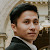Approximate Primal-Dual Method for Markov Random Fields Optimization

1 post in this topic

In this topic we will discuss Nikos Komodakis's work on the approximate primal-dual method for solving MRF optimization. I will describe in this topic 4 algorithms: PD1, PD2, PD3 and FastPD.

The MRF optimization (or MAP Inference) problem:
\begin{equation}
\begin{aligned}
&\text{minimize}  \quad    && \sum_{p\in V}\sum_{l\in L} \theta_p(l)x_p(l) + \sum_{pq\in E}\sum_{(l,l')\in L^2} \theta_{pq}(l,l')x_p(l)x_q(l')\\
&\text{subject to} \quad    && \sum_{l\in L} x_p(l) = 1 \quad \forall p\in V,\\
& &&\sum_{l'\in L} x_{pq}(l,l') = x_p(l) \quad\forall pq\in E, l\in L, \\
& &&\sum_{l\in L} x_{pq}(l,l') = x_q(l') \quad\forall pq\in E, l'\in L, \\
& &&x_p(l)\in\set{0,1}, x_{pq}(l,l')\in\set{0,1}\quad\forall pq\in E, l\in L, l'\in L.
\end{aligned}
\end{equation}

Replacing the integral constraints in the above problem by $x_p(l)\ge 0, x_{pq}(l,l')\ge 0$ we get its the LP-relaxation. The dual of this LP-relaxation is given by:

\begin{align}
\mbox{maximize}\quad & \sum_{p\in V}\min_{a\in L}h_p(a) \label{eq:dual-objective}\\
\mbox{subject to}\quad &y_{pq}(a) + y_{qp}(b) \le \theta_{pq}(a,b) \quad \forall pq\in E, a\in L,b\in L, \label{eq:dual-feasibility}\end{align}
where the function $h_p(\cdot)$ is defined by $$h_p(a) = \theta_p(a) + \sum_{q:pq\in E} y_{pq}(a).$$

Denote by $x_p$ the chosen label for the node $p$.

...

PD1 Algorithm

This algorithm, at termination, gives a primal-dual pair $(\mathbf{x},\mathbf{y})$ satisfying ($\mathbf{x}$ is always chosen as a labeling, thus primal feasibility always holds and hereafter we will not state it explicitly) :

1. Relaxed dual feasibility:
\begin{equation}y_{pq}(a) \le \frac{1}{2}\theta_{pq}^{\mathrm{min}}\quad\forall pq\in E,a\in L. \label{eq:relaxed-dual-feasibility} \end{equation}
Clearly, this implies dual feasibility, i.e. \eqref{eq:dual-feasibility}.
2. Relaxed complementary slackness conditions: \begin{align} h_p(x_p) &= \min_ah_p(a) \quad \forall p\in V \label{eq:primal-csc-p} \\ y_{pq}(x_p) + y_{qp}(x_q) &\ge \frac{1}{f_\mathrm{app}} \theta_{pq}(x_p,x_q) \quad \forall pq\in E. \label{eq:relaxed-primal-csc-pq} \end{align}

During its iterations, the algorithm ensures all the above conditions, except \eqref{eq:primal-csc-p}, which will be satisfied at the end.

Dual variables update

Given $(\mathbf{x},\mathbf{y})$ satisfying \eqref{eq:relaxed-dual-feasibility} and \eqref{eq:relaxed-primal-csc-pq}. If $(\mathbf{x},\mathbf{y})$ also satisfy \eqref{eq:primal-csc-p} then we obtain an approximate solution. Otherwise, we will be able to increase the dual objective \eqref{eq:dual-objective} as follows.

Since \eqref{eq:primal-csc-p} does not hold for all $p$, there exists a non-empty set $V_0 \subseteq V$ of nodes $p$ where $h_p(x_p) > \min_ah_p(a)$.

Create an account or sign in to comment

You need to be a member in order to leave a comment

Create an account

Sign up for a new account in our community. It's easy!

Register a new account

• Related Content

• By Khue
In this topic I would like to discuss everything about Linear Assignment Problems (LAPs). Another important class of assignment problems, that is Quadratic Assignment Problems, will be discussed later in another topic.
Let's begin with the classic LAP, defined as follows (Wikipedia):
This problem is also called Linear Sum Assignment Problem (LSAP), since the objective function is a sum, to be differentiated with other types of assignment problems such as the Linear Bottleneck Assignment Problem (LBAP). These problems will also be discussed later in this topic. When we say LAP without specification, we refer to the LSAP.
The formal mathematical definition of the LAP is the following.
Problem. (LAP) Given a matrix $C = (c_{ij})_{1\le i,j \le n} \in \mathbb{R}^{n\times n}$, called cost matrix, where $n$ is a given positive integer.
\begin{align} \mbox{minimize}\quad & \sum_{i=1}^n \sum_{j=1}^n c_{ij}x_{ij} \\ \mbox{subject to}\quad & \sum_{i=1}^n x_{ij} = 1 \quad \forall j, \\ & \sum_{j=1}^n x_{ij} = 1 \quad \forall i,\\ & x_{ij} \in \set{0,1} \quad\forall i,j. \end{align}
It is straightforward to see that this problem is equivalent to finding a perfect matching of a bipartite graph, thus it is also known as the bipartite minimum cost perfect matching problem.
It is well-known that the LP relaxation of the above integer program (i.e. the integral constraints $x_{ij} \in \set{0,1}$ are relaxed to $x_{ij} \ge 0$) has optimal solutions at extreme points, thus the LP relaxed problem and the original integer program are equivalent.
• By Khue
A matching $M$ in an undirected graph $G=(V,E)$ is a set of disjoint edges. A vertex $v$ that is not incident to any edge in $M$ is said to be exposed. Otherwise $v$ is matched. Given a graph $G=(V,E)$. The Maximum Matching Problem is to find a maximum (cardinality) matching $M$ of $G$.

The image shows an example of a matching with cardinality $3$, the three edges are $(1,6), (2,7)$ and $(3,8)$. The vertices $4,5,9$ and $10$ are exposed.
An alternating path with respect to $M$ (or an $M$-alternating path) is a path that alternates between edges in $M$ and edges in $E\setminus M$. An augmenting path with respect to $M$  (or an $M$-augmenting path) is a path in which the first and last vertices are exposed.
Theorem. A matching $M$ in a graph $G$ is maximum if and only if there is no $M$-augmenting path in $G$.
It is not difficult to prove the above theorem using the following lemma.
Lemma. If $M$ is a matching in $G$ and $P$ is an $M$-augmenting path then $M' := M\triangle P$ (which is the symmetric difference of $M$ and $P$, defined by $M\triangle P = (M\cup P)\setminus (M\cap P)$) is also a matching and $|M'|=|M|+1$.
By the above theorem, the problem of matching can be reduced to the problem of finding an augmenting path with respect to a given matching $M$.
We will first discuss the matching problem for bipartite graphs before moving onto general graphs. A graph $G=(V\cup U,E)$, $V$ and $U$ are disjoint, is called bipartite if every edge in $E$ has one endpoint in $V$ and the other in $U$.
We present an algorithm that searches for an $M$-augmenting path (if such a path exists), given a bipartite graph $G$ and a matching $M$ in it:
Begin with an exposed node $v\in V$. Find each neighbor $u$ of $v$: If $u$ is exposed, then we have found an augmenting path (that is $(v,u)$); If $u$ is not exposed, then denote by $v'$ its mate (i.e. $(u,v')$ is a matched edge). Continue the search with $v'$, by ignoring the vertices already encountered previously. During the search, if we encounter an exposed vertex, then we have found an augmenting path. If we do not encounter any exposed vertex, then the given matching is already maximum.
References
     A. Schrijver, Combinatorial Optimization: Polyhedra and Efficiency, Springer 2012.
     C. H. Papadimitriou and K. Steiglitz, Combinatorial Optimization: Algorithms and Complexity, Dover 1998.